# Maharashtra Board Class 11 Chemistry Solutions Chapter 12 Chemical Equilibrium

Balbharti Maharashtra State Board 11th Chemistry Textbook Solutions Chapter 12 Chemical Equilibrium Textbook Exercise Questions and Answers.

## Maharashtra State Board 11th Chemistry Solutions Chapter 12 Chemical Equilibrium

1. Choose the correct option

Question A.
The equilibrium, H2O(l) ⇌ H+(aq) + OH(aq) is
a. dynamic
b. static
c. physical
d. mechanical
a. dynamic

Question B.
For the equilibrium, A ⇌ 2B + Heat, the number of ‘A’ molecules increases if
a. volume is increased
b. temperature is increased
d. concentration of B is decreased
b. temperature is increased

Question C.
For the equilibrium Cl2(g) + 2NO(g) ⇌ 2NOCl(g) the concentration of NOCl will increase if the equilibrium is disturbed by ………..
b. removing NO
d. removal of Cl2

Question D.
The relation between Kc and Kp for the reaction A(g) + B(g) ⇌ 2C(g) + D(g) is
a. Kc = Kp/RT
b. Kp = Kc2
c. Kc = $$\frac{1}{\sqrt{\mathrm{Kp}}}$$
d. Kp/Kc = 1
a. Kc = Kp/RT

Question E.
When volume of the equilibrium reaction C(s) + H2O(g) ⇌ CO(g) + H2(g) is increased at constant temperature the equilibrium will
a. shift from left to right
b. shift from right to left
c. be unaltered
d. can not be predicted
a. shift from left to rightQuestion A.
State Law of Mass action.
Law of mass action: The law of mass action states that the rate of a chemical reaction at each instant is proportional to the product of concentrations of all the reactants.

Question B.
Write an expression for equilibrium constant with respect to concerntration.
For a reversible chemical reaction at equilibrium, aA + bB ⇌ cD + dD
Equilibrium constant (Kc) = $$\frac{[C]^{\mathrm{c}}[\mathrm{D}]^{\mathrm{d}}}{[\mathrm{A}]^{\mathrm{a}}[\mathrm{B}]^{\mathrm{b}}}$$

Question C.
Derive mathematically value of Kp for A(g) + B(g) ⇌ C(g) + D(g).
When the concentrations of reactants and products in gaseous reactions are expressed in terms of their partial pressure, then the equilibrium constant is represented as Kp.
∴ For the reaction,
A(g)+ B(g) ⇌ C(g) + D(g)
the equilibrium constant (KC) can be expressed using partial pressure as: Kp = $$\frac{P_{C} \times P_{D}}{P_{A} \times P_{B}}$$
Where PA, PB, PC and PD are equilibrium partial pressures of A, B, C and D respectively.

Question D.
Write expressions of KC for following chemical reactions
i. 2SO2(g) + O2(g) ⇌ 2SO3(g)
ii. N2O4(g) ⇌ 2NO2(g)
i. 2SO2(g) + O2(g) ⇌ 2SO3(g)
Kc = $$\frac{\left[\mathrm{SO}_{3}\right]^{2}}{\left[\mathrm{SO}_{2}\right]^{2}\left[\mathrm{O}_{2}\right]}$$

ii. N2O4(g) ⇌ 2NO2(g)
Kc = $$\frac{\left[\mathrm{NO}_{2}\right]^{2}}{\left[\mathrm{~N}_{2} \mathrm{O}_{4}\right]}$$Question E.
Mention various applications of equilibrium constant.
Various applications of equilibrium constant:

• Prediction of the direction of the reaction
• To know the extent of the reaction
• To calculate equilibrium concentrations
• Link between chemical equilibrium and chemical kinetics

Question F.
How does the change of pressure affect the value of equilibrium constant ?
The change of pressure does not affect the value of equilibrium constant.

Question J.
Differentiate irreversible and reversible reaction.
Irreversible reaction:

1. Products are not converted back to reactants.
2. Reaction stops completely and almost goes to completion.
3. It can be carried out in an open or closed vessel.
4. It takes place only in one direction. It is represented by →
5. e.g. C(s) + O2(g) → CO2(g)

Reversible reaction:

1. Products arc converted back to reactants.
2. Reaction appears to have stopped but does not undergo completion.
3. It is generally carried out in a closed vessel.
4. It takes place in both directions. It is represented by ⇌
5. e.g. N2(g) + O2(g) ⇌ 2NO(g)

Question K.
Write suitable conditions of concentration, temperature and pressure used during manufacture of ammonia by Haber process.
i. Concentration: Addition of H2 or N2 both favours forward reaction. This increases the yield of NH3.
ii. Temperature: The formation NH3 is exothermic. Hence, low temperature should favour the formation of NH3. However, at low temperatures, the rate of reaction is small. At high temperatures, the reaction occurs rapidly but decomposition of NH3 occurs. Hence, optimum temperature of about 773 K is used.
iii. Pressure: The forward reaction is favoured with high pressure as it proceeds with decrease in number of moles. At high pressure, the catalyst becomes inefficient. Therefore, optimum pressure needs to be used. The optimum pressure is about 250 atm.

Question L.
Relate the terms reversible reactions and dynamic equilibrium.

• Reversible reactions are the reactions which do not go to completion and occur in both the directions simultaneously.
• If such a reaction is allowed to take place for a long time, so that the concentrations of the reactants and products do not vary with time, then the reaction will attain equilibrium.
• Since, both the forward and backward reactions continue to take place in opposite directions in the same speed, the equilibrium achieved is dynamic in nature.

Thus, if the reaction is not reversible then it cannot attain dynamic equilibrium.

Question M.
For the equilibrium.
$$\mathrm{BaSO}_{4(\mathrm{~s})} \rightleftharpoons \mathrm{Ba}_{(\mathrm{aq})}^{2+}+\mathrm{SO}_{4(\mathrm{aq})}^{2-}$$
state the effect of
b. Removal of SO42- ion
on the equilibrium.
a. Addition of Ba2+ ion will favour the reverse reaction, (that is, equilibrium shifts from right to left). This increases the amount of BaSO4.
b. Removal of $$\mathrm{SO}_{4}^{2-}$$ ion will favour the forward reaction, (that is, equilibrium shifts from left to right). This decreases the amount of BaSO4.
c. Addition of BaSO4(s) will not affect the equilibrium as the equilibrium constant expression does not include pure solids.3. Explain :

Question A.
Dynamic nature of chemical equilibrium with suitable example.
Dynamic nature of chemical equilibrium:
i. Consider a chemical reaction: A ⇌ B.
Kc = [B]/[A]
At equilibrium, the ratio of concentration of the product to that of the concentration of the reactant is constant and this is equal to Kc.

ii. At this stage reaction takes place in both the directions with same speed although the reaction appears to have stopped. Thus, the chemical equilibrium is dynamic in nature. Dynamic means moving and at a microscopic level, the system is in motion.

iii. For example, in the reaction between H2 and I2 to form HI, the colour of the reaction mixture becomes constant because the concentrations of H2, I2 and HI become constant at equilibrium.
H2 + I2 ⇌ 2HI
Thus, when equilibrium is reached, the reaction appears to have stopped. However, this is not the case. The reaction is still going on in the forward and backward direction but the rate of forward reaction is equal to the rate of backward reaction. Hence, chemical equilibrium is dynamic in nature and not static.

Question B.
Relation between Kc and Kp.
Consider a general reversible reaction:
aA(g) + bB(g) ⇌ cC(g) + dD(g)
The equilibrium constant (Kp) in terms of partial pressure is given by equation:
Kp = $$\frac{\left(P_{C}\right)^{c}\left(P_{D}\right)^{d}}{\left(P_{A}\right)^{a}\left(P_{B}\right)^{b}}$$ …………(1)
For a mixture of ideal gases, the partial pressure of each component is directly proportional to its concentration at constant temperature.
For component A,
PAV = nART
PA = $$\frac{\mathrm{n}_{\mathrm{A}}}{\mathrm{V}}$$ × RT
$$\frac{\mathrm{n}_{\mathrm{A}}}{\mathrm{V}}$$ is molar concentration of A in mol dm-3 V
∴ PA = [A]RT where, [A] = $$\frac{\mathrm{n}_{\mathrm{A}}}{\mathrm{V}}$$
Similarly, for other components, PB = [B]RT, PC = [C]RT, PD = [D]RT
Now substituting equations for PA, PB, PC, PD in equation (1), we get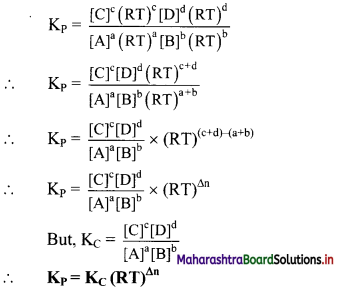where Δn = (number of moles of gaseous products) – (number of moles of gaseous reactants) in the balanced chemical equation.
R = 0.08206 L atm K-1 mol-1
[Note: While calculating the value of Kp, pressure should be expressed in bar, because standard state of pressure is 1 bar. 1 pascal (Pa) = 1 N m-2 and 1 bar = 105 Pa]

Question C.
State and explain Le Chatelier’s principle with reference to
1. change in temperature
2. change in concerntration.
Statement: When a system at equilibrium is subjected to a change in any of the factors determining the equilibrium conditions of a system, system will respond in such a way as to minimize the effect of change.

1. Change in temperature:

• Consider the equilibrium reaction,
PCl5(g) ⇌ PCl3(g) + Cl2(g) + 92.5 kJ
• The forward reaction is exothermic. According to Le Chatelier’s principle an increase in temperature shifts the position of equilibrium to the left.
• The reverse reaction is endothermic. An endothermic reaction consumes heat. Therefore, the equilibrium must shift in the reverse direction to use up the added heat (heat energy converted to chemical energy).
• Thus, an increase in temperature favours formation of PCl5 while a decrease in temperature favours decomposition of PCl5.

2. Change in concentration:

• Consider reversible reaction representing production of ammonia (NH3).
N2(g) + 3H2(g) ⇌ 2NH3(g) + Heat
• According to Le Chatelier’s principle, when H2 or N2 is added to equilibrium, the effect of addition of H2 or N2 or is reduced by shifting the equilibrium from left to right so that the added N2 or H2 is consumed.
• The forward reaction occurs to a large extent than the reverse reaction until the new equilibrium is established. As a result, the yield of NH3 is increases.
• In general, if the concentration of one of the species in equilibrium mixture is increased, the position of equilibrium shifts in the opposite so as to reduce the concentration of this species. However, the equilibrium constant remains unchanged.Question D.
a. Reversible reaction
b. Rate of reaction
a. Reversible reaction:
i. Reactions which do not go to completion and occur in both the directions simultaneously are called reversible reactions.
ii. Reversible reactions proceed in both directions. The direction from reactants to products is the forward reaction, whereas the opposite reaction from products to reactants is called the reverse or backward reaction.
iii. A reversible reaction is denoted by drawing in between the reactants and product a double arrow, one pointing in the forward direction and other in the reverse direction (⇌ or ⇄).
ii. At high temperature in an open container, the CO2 gas formed will escape away. Therefore, it is not possible to obtain back
e.g. a. H2(g) + I2(g) ⇌ 2HI(g)
b. CH3COOH(aq) + H2O(l) ⇌ CH3COO(aq) + H3O+(aq)

b. Rate of reaction:
Rate of a chemical reaction:
i. The rate of a chemical reaction can be determined by measuring the extent to which the concentration of a reactant decreases in the given time interval, or extent to which the concentration of a product increases in the given time interval.
ii. Mathematically, the rate of reaction is expressed as:
Rate = $$-\frac{\mathrm{d}[\text { Reactant }]}{\mathrm{dT}}=\frac{\mathrm{d}[\text { Product }]}{\mathrm{dT}}$$
where, d[reactant] and d[product] are the small decrease or increase in concentration during the small time interval dT.

Question E.
What is the effect of adding chloride on the position of the equilibrium ?
AgCl(s) ⇌ Ag+(aq) + Cl(aq)
Addition of Cl ion will favour the reverse reaction, (that is, equilibrium shift from right to left) This increases the amount of AgCl.

11th Chemistry Digest Chapter 12 Chemical Equilibrium Intext Questions and Answers

Can you recall? (Textbook Page No. 174)

Question 1.
What are the types of the following changes?
Natural waterfall, spreading of smoke from burning incense stick, diffusion of fragrance of flowers.
Answer: Natural waterfall, spreading of smoke from burning incense stick and diffusion of fragrance of flowers are irreversible physical changes.

Try this. (Textbook Page No. 174)

Question 1.
Dissolve 4 g cobalt chloride in 40 mL water. It forms a reddish pink solution. Add 60 mL concentrated HCl to this. It will turn blue. Take 5 mL of this solution in a test tube and place it in a beaker containing ice water mixture. The colour of solution will become pink. Place the same test tube in a beaker containing water at 90 °C. The colour of the solution turns blue.
Inference: The colour change of the solution from pink to blue is caused by the chemical reaction. On changing the temperature, the direction of the reaction reverses. This indicates that the chemical reaction is reversible. This activity is an example of a reversible chemical reaction.
The reaction can be written as: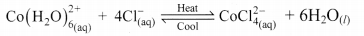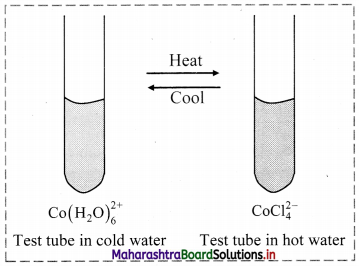Can you tell? (Textbook Page No. 174)

Question 1.
What does violet colour of the solution in the activity mentioned in Q.2 indicate?
In the reaction, the reactant $$\mathrm{Co}\left(\mathrm{H}_{2} \mathrm{O}\right)_{6}^{2+}$$ is pink in colour and the product $$\mathrm{CoCl}_{4}^{2-}$$ is blue in colour. When the solution contains both the reactant and product, the resulting solution will appear violet. This indicates that the reaction has attained equilibrium (that is, the reaction proceeds in both the direction with equal rates and is a reversible reaction).(Textbook Page No. 174)

Question 1.
Calcium earbonate when heated strongly, decomposes to form calcium oxide and carbon dioxide.
i. If this reaction is carried out in a closed container, what will we observe?
ii. Consider this reaction occurring in an open system or container, what will happen? Can we obtain back calcium carbonate?
At high temperature in a closed container, we will find that after certain time, some calcium carbonate is present. If we continue the experiment over a longer period of time at the same temperature, the concentrations of calcium carbonate, calcium oxide and carbon dioxide remain unchanged. The reaction thus appears to have stopped and the system has attained the equilibrium. Actually, the reaction does not stop but proceeds in both the directions with equal rates. In other words, calcium carbonate decomposes to give calcium oxide and carbon dioxide at a particular rate. Exactly at the same rate the calcium oxide and carbon dioxide recombine and form calcium carbonate. Thus, in closed container, reversible reaction occurs.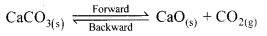ii. At high temperature in an open container, the CO2 gas formed will escape away. Therefore, it is not possible to obtain back calcium carbonate. Thus, in an open container, irreversible reaction occurs.
$$\mathrm{CaCO}_{3(\mathrm{~s})} \stackrel{\text { Heat }}{\longrightarrow} \mathrm{CaO}_{(\mathrm{s})}+\mathrm{CO}_{2(\mathrm{~g})}$$

Internet my friend (Textbook Page No. 175)

Question 1.
i. Equilibrium existing in the formation of oxyhaemoglobin in human body
ii. Refrigeration system in equilibrium
i. Equilibrium existing in the formation of oxyhaemoglobin in human body:
Oxygen is transported in the body with the assistance of red blood cells. The red blood cells contain a pigment called haemoglobin. Each haemoglobin molecule binds four oxygen molecules to form oxyhaemoglobin. Thus, the oxygen molecules are carried to individual cells in the body tissue where they are released.

The binding of oxygen to haemoglobin is a reversible reaction.
Hb + 4O2 ⇌ Hb.4O2
When the oxygen concentration is high (in the lungs), haemoglobin and oxygen combine to form oxyhaemoglobin and the reaction achieves equilibrium. But, when the oxygen concentration is low (in the body tissue), the reverse reaction occurs, that is, oxyhaemoglobin dissociates to haemoglobin and oxygen.
Thus, an equilibrium exists in the formation of oxyhaemoglobin in the human body.

ii. Refrigeration system in equilibrium:
a. Refrigeration system works on the principle of thermal equilibrium i.e., when a cold body comes in contact with a hot body then the heat flows from hot body to cold body until both the bodies attain the same temperature.
b. In the same way, a liquid (called as refrigerant) passes through the various compartments in the refrigerator and eventually lowers the temperature inside the refrigerator. This cycle is briefly described below:
Refrigerant flows through the compressor, which raises the pressure of the refrigerant. Next, the refrigerant flows through the condenser, where it condenses from vapor form to liquid form, giving off heat in the process. The heat given off is what j makes the condenser “hot to the touch.” After the condenser, the refrigerant goes through the expansion valve, where it experiences a pressure drop. Finally, the refrigerant goes to the evaporator. The refrigerant draws heat from the evaporator which causes the refrigerant to vaporize. The evaporator draws heat from the region that is to be cooled. The vaporized refrigerant goes back to the compressor to restart the cycle. In each of the heat transfer process, equilibrium is achieved (that is, heat given off is equivalent to the cooling achieved.)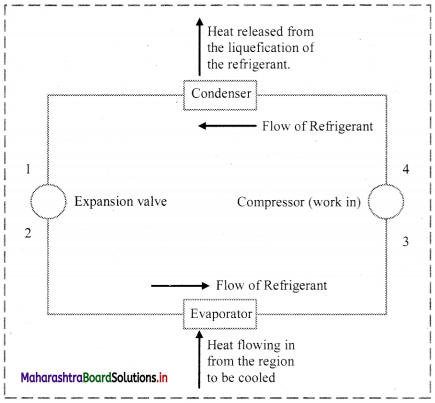[Note: Students are expected to collect additional information about equilibrium existing in the formation of oxyhaemoglobin in human body’ and ‘refrigeration system in equilibrium on their own.]

Try this. (Textbook Page No. 176)

Question 1.
i. Place some iodine crystals in a closed vessel. Observe the change in colour intensity in it.
ii. What do you see in the flask after some time?
i. The vessel gets slowly filled up with violet coloured vapour of iodine. After a certain time, the intensity of violet colour becomes stable.
ii. After sometime, both solid iodine and iodine vapour are present in the closed vessel. Iodine crystals will be seen deposited near the mouth of the flask and violet coloured vapour will be filled in the entire flask. It means solid iodine sublimes to give iodine vapour and the iodine vapour condenses to form solid iodine. The stable intensity of the colour indicates a state of equilibrium between solid and vapour iodine.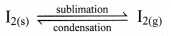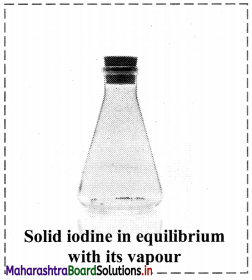Try this. (Textbook Page No. 176)

Question 1.
i. Dissolve a given amount of sugar in minimum amount of water at room temperature.
ii. Increase the temperature and dissolve more amount of sugar in the same amount of water to make a thick sugar syrup solution.
iii. Cool the syrup to the room temperature.
Observation: Sugar crystals separate out.
Inference: The sugar syrup solution prepared is a saturated solution. Therefore, additional amount of sugar cannot be dissolved in it at room temperature.
In a saturated solution, there exists dynamic equilibrium between the solute molecules in the solid state and in dissolved state.
Sugar(aq) ⇌ Sugar(s)
The rate of dissolution of sugar = The rate of crystallization of sugar.
However, when it is heated, additional amount of sugar can be dissolved in it. But when such a thick sugar syrup is cooled again to room temperature, sugar crystals separate out.Do you know? (Textbook Page No. 177)

Question 1.
What is a saturated solution?
A saturated solution is the solution when additional solute cannot be dissolved in it at the given temperature. The concentration of solute in a saturated solution depends on temperature.

Observe and discuss. (Textbook Page No. 177)

Question 1.
Colourless N2O4 taken in a closed flask is converted to NO2 (a reddish brown gas).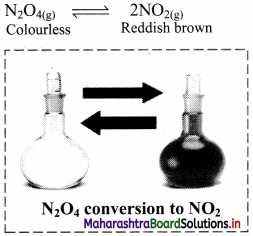Observation: Initially, the colourless gas (N2O4) turns to reddish brown (NO2) gas. After sometime, the colour becomes lighter indicating the formation of N2O4 from NO2.
Inference: This indicates that the reaction is reversible. In such reaction, the reactants combine to form the products and the products combine to give the reactants. As soon as the forward reaction produces any NO2, the reverse reaction begins and NO2, starts combining back to N2O4. At equilibrium, the concentrations of N2O4 and NO2 remain unchanged and do not vary with time, because the rate of formation of NO2 is equal to the rate of formation of N2O4.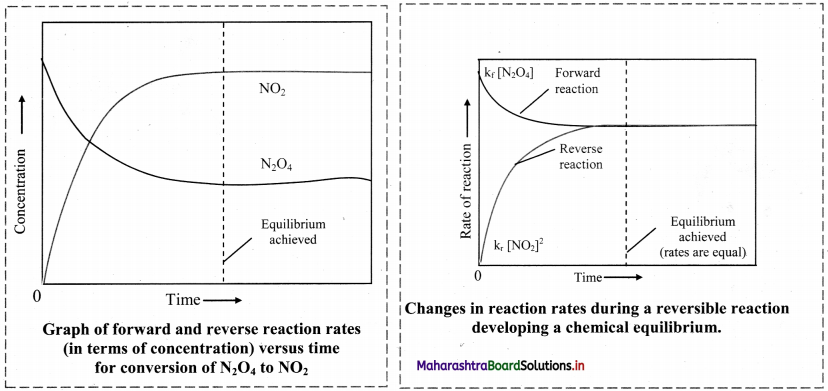[Note: For any reversible reaction in a closed system whenever the opposing reactions (forward and reaction) are occurring at different rates, the forward reaction will gradually become slower and the reverse reaction will become faster. Finally, the rates become equal and equilibrium is established.]

Discuss (Textbook Page No. 177)

i. Consider the following dissociation reaction: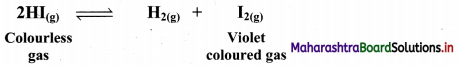The reaction is carried out in a closed vessel starting with hydrogen iodide.
ii. Now, let us start with hydrogen and iodine vapour in a closed container at a certain temperature.
H2(g) + I2(g) ⇌ 2HI(g)
i. Starting with hydrogen iodide:
Observations:
a. At first, there is an increase in the intensity of violet colour.
b. After certain time, the increase in the intensity of violet colour stops.
c. When contents in a closed vessel are analyzed at this stage, it is observed that reaction mixture contains the hydrogen iodide, hydrogen and iodine with their concentrations being constant over time.
Inference:
The rate of decomposition of HI becomes equal to the rate of combination of H2 and I2. At equilibrium, no net change is observed and both reactions continue to occur at equal rates.
Thus, the reaction represents chemical equilibrium.

ii. Starting with hydrogen and iodine:
Observations:
a. At first, there is a decrease in the intensity of violet colour.
b. After certain time, the decrease in the intensity of violet colour stops.
c. When contents in a closed vessel are analyzed at this stage, it is observed that reaction mixture contains hydrogen, iodine and hydrogen iodide with their concentrations being constant over time.
Inference:
The rate of combination of H2 and I2 becomes equal to the rate of decomposition of HI. The reaction attains chemical equilibrium.Can you recall? (Textbook Page No. 180)

Question 1.
Write ideal gas equation with significance of each term involved in it.
Ideal gas equation is PV = nRT.
where, P = Pressure of the gas
V = Volume of the gas
n = Number of moles of the gas
R = universal gas constant
T = Absolute temperature of the gas

Just think. (Textbook page no. 181)

Question 1.
Two processes, which are taking place in opposite directions are in equilibrium. How to write equilibrium constant expersions for heterogeneous equilibrium?
Equilibrium in a system having more than one phase is called heterogeneous equilibrium.
If ethanol is placed in a conical flask, liquid-vapour equilibrium is established.
C2H5OH(l) ⇌ C2H5OH(g)
For a given temperature,
Kc = $$\frac{\left[\mathrm{C}_{2} \mathrm{H}_{5} \mathrm{OH}_{(g)}\right]}{\left[\mathrm{C}_{2} \mathrm{H}_{5} \mathrm{OH}_{(l)}\right]}$$
But [C2H5OH(l)] = 1
∴ Kc = [C2H5OH(g)]
Thus, at any given temperature, density is constant irrespective of the amount of liquid, and the term in the denominator is also constant.
ii. similarly, consider I2(g) ⇌ I2(g)
Kc = [I2(g)]
iii. Thus, the expression for equilibrium constant does not contain the concentration of pure solids and pure liquids. That is because for any pure liquid and solid, the concentration is simply its density and this will not change no matter how much solid or liquid is used. Hence, the expression for heterogeneous equilibrium only uses the concentration of gases and dissolved substances (aq.). Solids are pure substances with unchanging concentrations and thus equilibria including solids are simplified.

Can you tell? (Textbook Page No. 183)

Question 1.
Comment on the extent to which the forward reaction will proceed, from the magnitude of the equilibrium constant for the following reactions:
i. H2(g) + I2(g) ⇌ 2HI(g), Kc = 20 at 550 K
ii. H2(g) + Cl2(g) ⇌ 2HCl(g), Kc = 1018 at 550 K
i. For the reaction, Kc = 20 at 550 K
If the value of Kc is the range of 10-3 to 103, the forward and reverse proceed to equal extents.
Hence, the given reaction will form appreciable concentrations of both reactants and the product at equilibrium.

ii. For the reaction, Kc = 1018 at 550 K
If the value of Kc >>> 103, forward reaction is favoured.
Hence, the given reaction will proceed in the forward direction and will nearly go to completion.

Use your brain power (Textbook Page No. 183)

Question 1.
The value of Kc for the dissociation reaction:
H2(g) ⇌ 2H(g) is 1.2 × 10-42 at 500 K.
Does the equilibrium mixture contain mainly hydrogen molecules or hydrogen atoms?
When the value of Kc is very low (that is, Kc < 10-3), then at equilibrium, only a small fraction of the reactants is converted into products.
For the given reaction, Kc <<< 103 at 500 K.
Hence, the equilibrium mixture contains mainly hydrogen molecules.Internet my friend (Textbook Page No. 183)

Question 1.
https://www.chemguide.co.uk/physical/equilibria/introduction.html
[Note: Students can use the above link as reference and collect information about chemical equilibrium.]

Can you tell? (Textbook Page No. 188)

i. If NH3 is added to the equilibrium system (Haber process), in which direction will the equilibrium shift to consume added NH3 to reduce the effect of stress?
ii. In this process, out of the reactions (reverse and forward reaction), which reaction will occur to a greater extent?
iii. What will be the effect on yield of NH3?